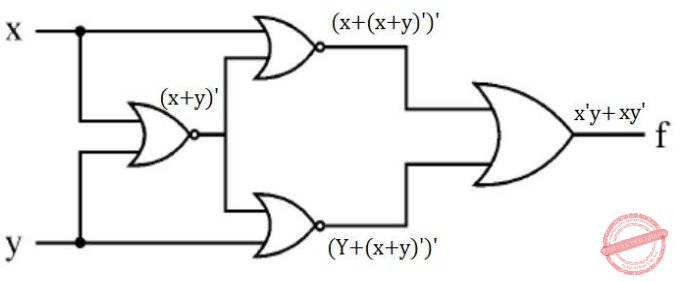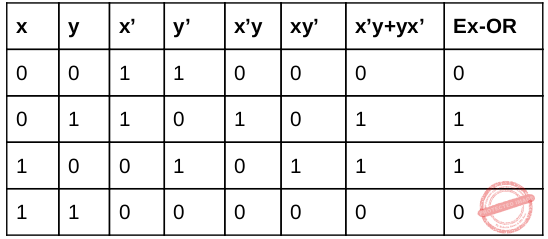## UGC NET CS 2016 Aug- paper-2

 Question 1
The Boolean function [~(~p ∧ q) ∧ ~( ~p ∧ ~q)] ∨ (p ∧ r) is equal to the Boolean function:
 A q B p ∧ r C p ∨ q D p
Digital-Logic-Design       Boolean-Algebra
Question 1 Explanation:Question 2
Let us assume that you construct ordered tree to represent the compound ​ proposition (~ (p ∧ q)) ↔ (~ p ∨ ~ q) Then, the prefix expression and post-fix​ expression determined using this ordered tree are given as ____ and _____ respectively.
 A ↔~∧pq∨ ~ ~ pq, pq∧~p~q~∨↔ B ↔~∧pq∨ ~ p~q, pq∧~p~q~∨↔ C ↔~∧pq∨ ~ ~ pq, pq∧~p~~q∨↔ D ↔~∧pq∨ ~ p~ q, pq∧~p~ ~q∨↔
Algorithms       Trees
Question 2 Explanation:
Step-1: Given compound proposition is
(~(p ∧ q))↔(~ p ∨ ~ q)
It is clearly specifying that
↔ is a root
(~(p ∧ q)) is left subtree
(~p ∨ ~q) is right subtree.
Step-2: Finally the tree is looks likeStep-3: Prefix operation traverse through Root,left and Right ↔~∧pq∨ ~ p~q Step-4: Postfix operation traverse through Left,Right and Root.
pq∧~p~q~∨↔
 Question 3
Let A and B be sets in a finite universal set U. Given the following: |A – B|, |A ⊕ B|, |A| + |B| and |A ∪ B| Which of the following is in order of increasing size ?
 A |A – B| ≤ |A ⊕ B| ≤ |A| + |B| ≤ |A ∪ B| B |A ⊕ B| ≤ |A – B| ≤ |A ∪ B| ≤ |A| + |B| C |A ⊕ B| ≤ |A| + |B| ≤ |A – B| ≤ |A ∪ B| D |A – B| ≤ |A ⊕ B| ≤ |A ∪ B| ≤ |A| + |B|
Engineering-Mathematics       Set-Theory
Question 3 Explanation:
Step-1: Let A and B be sets in a finite universal set U.
Step-2: |A–B| we can also write into |A|-|A ∩ B| and equivalent Venn diagram isStep-3: |A⊕B| We can also write into |A|+|B|- 2|A∩B| and equivalent Venn diagram isStep-4: |A| + |B| We can represented into |A| + |B| and equivalent Venn diagram isStep-5: |A∪B| We can also write into |A|+|B|- |A∩B| and equivalent Venn diagram isStep-6: |A – B| ≤ |A ⊕ B| ≤ |A ∪ B| ≤ |A| + |B| is correct order.
 Question 4
What is the probability that a randomly selected bit string of length 10 is a palindrome?
 A 1/64 B 1/32 C 1/8 D 1⁄4
Engineering-Mathematics       Probability
Question 4 Explanation:
Palindrome is a number that remains the same when its digits are reversed.
Ex: 0, 1, 2, 3, 4, 5, 6, 7, 8, 9, 11, 22, 33, 44, 55, 66, 77, 88, 99, 101, 111, 121...,
Step-1:​ Here, string length is 10. If we consider first 5 numbers as random choices like 0 or 1.
Remaining 5 numbers are fixed. Total number of possibilities are 2​ 10​ . But we are only considering first 5 choices. The probability is 2​ 5​ .
Step-2:​ The probability 2​ 5​ /2​ 10
= 1/2​ 5
= 1/32
 Question 5
Given the following graphs:Which of the following is correct?
 A G​ 1​ contains Euler circuit and G​ 2​ does not contain Euler circuit. B G​ 1​ does not contain Euler circuit and G​ 2​ contains Euler circuit. C Both G​ 1​ and G​ 2​ do not contain Euler circuit. D Both G​ 1​ and G​ 2​ contain Euler circuit.
Engineering-Mathematics       Graph-Theory
Question 5 Explanation:Step-1: G1 have odd number of vertices. So, it is not euler circuit.
Step-2: G2 also have odd number of vertices. So, it not euler circuit.
 Question 6
The octal number 326.4 is equivalent to
 A (214.2)​ 10​ and (D6.8))​ 16 B (212.5)​ 10​ ​ and (D6.8))​ ​ 16 C (214.5)​ 10​ and (D6.8))​ ​ 16 D (214.5)​ 10​ and (D6.4))​ ​ 16
Digital-Logic-Design       Number-Systems
Question 6 Explanation:
(326.4) 8 = ( ?)
Step1: First convert given octal no. to binary number because it will be easier to solve this way.Step 2: Now convert above binary no. into decimal .
(011010110.100) 2
= ( 1 * 2 7 ) + ( 1 × 2 6 ) + ( 1 × 2 4 ) + ( 1 × 2 2 ) + ( 1 × 2 1 ) · [ 1 × ( 1/2) ]
= (214.5) 10
(326.4) 8 = (?) 16 Step 1: Convert given octal no. into binary no.Step 2: Now convert above binary no. into decimal .
(011010110.100) 2
= ( 1 * 2 7 ) + ( 1 × 2 6 ) + ( 1 × 2 4 ) + ( 1 × 2 2 ) + ( 1 × 2 1 ) · [ 1 × ( 1/2) ]
= (214.5) 10
(326.4) 8 = (?) 16Question 7
Which of the following is the most efficient to perform arithmetic operations on the numbers?
 A Sign-magnitude B 1’s complement C 2’s complement D 9’s complement
Digital-Logic-Design       Number-Systems
Question 7 Explanation:
2’s complement has single representation for zero , but Sign-magnitude, 1’s complement and 9’s complement have two representations for 0 (i.e., both positive zero and negative zero). While doing arithmetic operations like addition or subtraction using 1's complement(or 9's complement), we have to add an extra carry bit, i.e 1 to the result to get the correct answer. 2's complement doesn't require such extra calculation.
 Question 8
The Karnaugh map for a Boolean function is given asThe simplified Boolean equation for the above Karnaugh Map is
 A AB + CD + AB’ + AD B AB + AC + AD + BCD C AB + AD + BC + ACD D AB + AC + BC + BCD
Digital-Logic-Design       K-Map
Question 8 Explanation:Question 9
Which of the following logic operations is performed by the following given combinational circuit?A EXCLUSIVE-OR B EXCLUSIVE-NOR C NAND D NOR
Digital-Logic-Design       Combinational-Circuits
Question 9 Explanation:Question 10
Match the following:A a-iii, b-ii, c-iv, d-i B a-ii, b-iv, c-i, d-iii C a-ii, b-i, c-iv, d-iii D a-iii, b-i, c-iv, d-ii
Digital-Logic-Design       Match-the-following
Question 10 Explanation:
a. Controlled Inverter : A circuit that transmits a binary word or its1’s complement.
b. Full adder : A circuit that can add 3 bits. It adds two data bits(ai,bi) and also carry bit(ci).
 Question 11
Given i= 0, j = 1, k = –1, x = 0.5, y = 0.0 What is the output of given ‘C’ expression ?
x * 3 && 3 || j | k
 A -1 B 0 C 1 D 2
C-Programming       Operator
Question 11 Explanation:
Step-1: Evaluate x ​ * 3 because multiplication has more priority than remaining operators x * 3→ 1.5
Step-2: && is logical AND. Both the statements are TRUE, then it returns 1 otherwise 0.
1.5 && 3 is TRUE. So, it returns 1.
Step-3: j | k is bitwise OR operator. It returns -1.
Step-4: ((x * 3) && 3) || (j | k) islogical OR operator. It returns 1
Note: The precedence is ((x * 3) && 3) || (j | k)
 Question 12
The following ‘C’ statement : int *f [ ] ( ); declares:
 A A function returning a pointer to an array of integers. B Array of functions returning pointers to integers. C A function returning an array of pointers to integers. D An illegal statement.
Programming       Programming
Question 12 Explanation:
int *f [ ] ( ); It declares array of functions returning pointers to integers.
There are 12 questions to complete.

Register Now